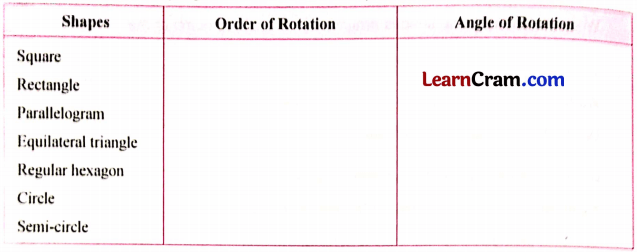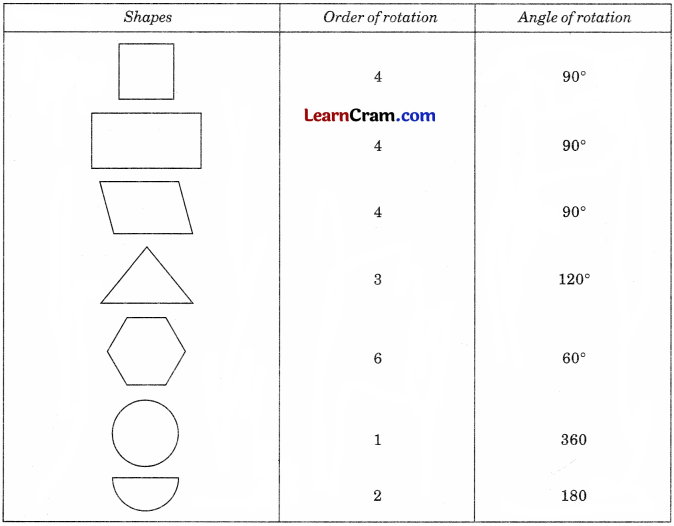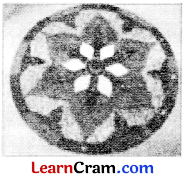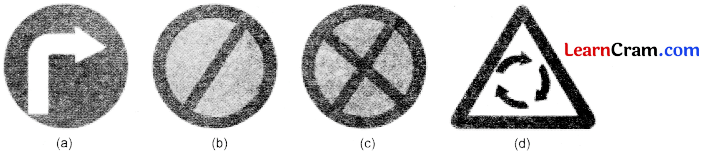# DAV Class 8 Maths Chapter 16 Worksheet 2 Solutions

The DAV Class 8 Maths Solution and DAV Class 8 Maths Chapter 16 Worksheet 2 Solutions of Rotational Symmetry offer comprehensive answers to textbook questions.

## DAV Class 8 Maths Ch 16 WS 2 Solutions

Question 1.
Name 2 figures having both line symmetry and rotational symmetry.
Solution:
Cricle and equilateral triangles have their rotational symmetry as well as line symmetry.

Question 2.
Make cutouts of the shapes given in Column I and complete the given table:Solution:### DAV Class 8 Maths Chapter 16 Value Based Questions

Question 1.
On the ocassion of Independence Day, a school organised a Rajigoli Competition. Mehak made a beautiful rangoli design and won the first prize in the competition.(i) Does rotational symmetry exist in the given design? If yes, then find the angle and order of rotation.
(ii) What is the importance of celebrating Independence Day?
Solution:
(i) Yes, the rotational symmetry exists in the given design.
If the given design is rotated about its centre, it repeats itself six times in a full turn.
So, it has a rotational symmetry of order 6.
Angle of rotation of the design = $$\frac{360^{\circ}}{6}$$ = 60°.

(ii) Celebrating Independence Day develops the spirit of unity among the citizens. It is also a way of tribute to martyrs.Question 2.
A school celebrated ‘Road Safety Week’ and the children made circular posters of various traffic signs. The teacher asked Rohan and Sohan to display all the posters on the bulletin board.(i) Which of these signs exhibit rotational symmetry? Also find their angle and order of rotation.
Solution:
(i) Signs (b), (c) and (d) exhibit rotational symmetry.
Angle of rotation of sign (b) = 180°
Order of rotation of sign (b) = 2
Angle of rotation of sign (c) = 90°
Order of rotation of sign (c) = 4
Angle of rotation of sign (d) = 120°
Order of rotation of sign (d) = 3

(ii) Write the meaning of these safety signs.
Solution:
(a) Compulsory right turn
(b) No parking
(c) No stopping